# Non Verbal Reasoning - Cubes and Dice

### Exercise :: Cubes and Dice - Section 2

31.

A cube has six different symbols drawn over its six faces. The symbols are dot, circle, triangle, square, cross and arrow. Three different positions of the cube are shown in figures X, Y, and Z.

Which symbol is opposite the dot?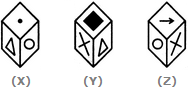A. Circle B. Triangle C. Arrow D. Cross

Answer: Option D

Explanation:

From figures X and Y, we conclude that dot, circle, square and cross lie adjacent to the triangle. Therefore, the arrow must lie opposite the triangle. From figures X and Z, we conclude that dot, triangle, arrow and cross lie adjacent to the circle. Therefore, the square must lie opposite the circle. Thus, the arrow lies opposite the triangle, the square lies opposite the circle and consequently, the cross lies opposite the dot.

As analysed above, the cross lies opposite the dot.

32.

How many dots are there on the dice face opposite the one with three dots?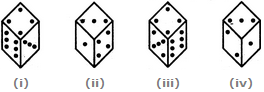A. 2 B. 4 C. 5 D. 6

Answer: Option C

Explanation:

From figures (i), (ii) and (iv), we conclude that 6, 4,1 and 2 dots appear adjacent to 3 dots. Clearly, there will be 5 dots on the face opposite the face with 3 dots.

33.

Two positions of a dice are shown below. When there are two dots at the bottom, the number of dots at the top will be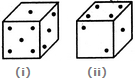A. 3 B. 5 C. 6 D. Cannot be determined

Answer: Option A

Explanation:

Number 1 is common to both the positions of the dice. We assume the dice in fig. (ii) to be rotated so that 1 dot moves to the top face (i.e. face V as per activity 1) i.e. to the same position as in fig. (i) and 2 and 4 dots move to the faces hidden behind the faces with 3 and 5 dots respectively. Thus, the combined figure will have 1 dot on the Top (i.e. on face V), 5 dots on Front face (i.e. on face I), 3 dots on RHS face (i.e. face II), 4 dots on the Rear face (i.e. face III) and 2 dots on the LHS face (i.e. face IV). Clearly, 3 dots lie on the face opposite the face having 2 dots. Therefore, when there are 2 dots at the bottom, the number of dots at the top will be 3.

34.

Two positions of a dice are shown below. If the face with 1 dot is at the bottom, then the number of dots on the top is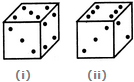A. 2 B. 3 C. 4 D. 5

Answer: Option B

Explanation:

From figures (i) and (ii), we conclude that 5, 4, 6 and 2 dots appear adjacent to 3 dots. Therefore, 1 dot must appear opposite 3 dots. Thus, if the face with 1 dot is at the bottom, then the face with 3 dots will appear on the top.

35.

Amongst the following figures, find the correct one, if it is known that the total number of dots on opposite faces of the cube shown is always 7.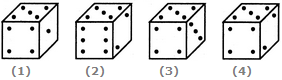A. Fig.1 B. Fig.2 C. Fig.3 D. Fig.4

Answer: Option A

Explanation:

Since the total number of dots on opposite faces is always 7, therefore 1 dot must lie opposite 6 dots, 2 dots must lie opposite 5 dots and 3 dots must lie opposite 4 dots. In each of the two figures (2) and (4), 2 dots appear adjacent to 5 dots, and in fig. (3), 3 dots appear adjacent to 4 dots. Hence, these figures are incorrect. Therefore/only fig. (1) is correct.

#### Current Affairs 2021

Interview Questions and Answers